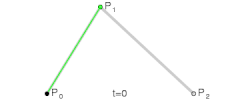## latex

HTML设计之初，没有考虑到数学公式排版，所以要想在里面添加一些比较复杂的符号就没有标签可以使用，唯一的折衷方法是，利用图片来代替显示，但是这样实在是麻烦。

MathML最大的弊端是，一个很简单的公式，用标签写起来要浪费一大堆文本，混杂在一起，没有第三方辅助工具的话，很难编辑和阅读。

wordpress也有相应的支持，提供一个生成latex图像的服务器，把要处理的latex文本作为url里的一个参数传入，就会返回一幅图片，http://l.wordpress.com/latex.php?latex=，后面这个latex=的参数就是latex源数据，只需要加入一个img标签，然后把src写成相应的url，就可支持数学公式的显示。当然，这样毕竟还是麻烦，有时候还要转换url编码，wordpress有一个latex插件，直接支持方括号latex标签，很方便。

[latex size=”2″]frac{x+sum_{i=0}^{n}{(i^2+int_a^b{3sin^i(x)dx}})}{sqrt{x^2+y^2}piepsilon}[/latex]

## bezier curve

Bezier曲线很常用，一般2D绘图软件里都有。比如photoshop，flash之类。

[latex] B(t)=P_0+t(P_1-P_0)=(1-t)P_0+tP_1,tin[0,1] [/latex][latex]Q_0=(1-t)P_0+tP_1[/latex]
[latex]Q_1=(1-t)P_1+tP_2[/latex]

[latex]B=(1-t)Q_0+tQ_1=(1-t)^2P_0+2t(1-t)P_1+t^2P_2[/latex]

t从0到1增加，就得到了一条曲线，如下图[latex]B(t)=(1-t)^3P_0+3(1-t)^2tP_1+3(1-t)t^2P_2+t^3P_3,tin[0,1][/latex]

[latex size=”2″]B(t)=sum_{i=0}^{n}{binom{n}{i}(1-t)^{n-i}t^iP_i}[/latex]

[latex size=”1″]=(1-t)^nP_0+binom{n}{1}(1-t)^{n-1}tP_1+..+t^nP_n,tin[0,1][/latex]